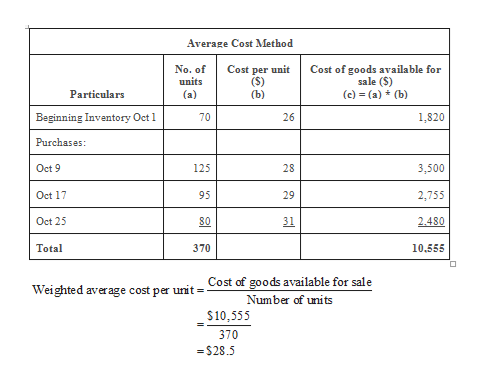# You have the following information for Waterway Industries for the month ended October 31, 2022. Waterway uses a periodic method for inventory.Date Description Units Unit Cost or Selling PriceOct. 1 Beginning inventory 70 \$26Oct. 9 Purchase 125 28Oct. 11 Sale 95 40Oct. 17 Purchase 95 29Oct. 22 Sale 70 45Oct. 25 Purchase 80 31Oct. 29 Sale 105 45 Calculate the weighted-average cost. (Round answer to 3 decimal places, e.g. 5.125.)Weighted-average cost per unit \$enter weighted-average cost per unit in dollars  Calculate ending inventory, cost of goods sold, gross profit under each of the following methods.(1) LIFO.(2) FIFO.(3) Average-cost. (Round answers to 0 decimal place, e.g. 125.)  LIFOFIFOAVERAGE-COSTThe ending inventory\$enter a dollar amount \$enter a dollar amount \$enter a dollar amount The cost of goods sold\$enter a dollar amount \$enter a dollar amount \$enter a dollar amount Gross profit\$enter a dollar amount \$enter a dollar amount \$enter a dollar amount

Question
40 views

You have the following information for Waterway Industries for the month ended October 31, 2022. Waterway uses a periodic method for inventory.

 Date Description Units Beginning inventory 70 \$26 Purchase 125 28 Sale 95 40 Purchase 95 29 Sale 70 45 Purchase 80 31 Sale 105 45

Calculate the weighted-average cost. (Round answer to 3 decimal places, e.g. 5.125.)

 Weighted-average cost per unit \$enter weighted-average cost per unit in dollars

Calculate ending inventory, cost of goods sold, gross profit under each of the following methods.

(1) LIFO.
(2) FIFO.
(3) Average-cost. (Round answers to 0 decimal place, e.g. 125.)

LIFO
FIFO
AVERAGE-COST
The ending inventory

\$enter a dollar amount \$enter a dollar amount \$enter a dollar amount

The cost of goods sold

\$enter a dollar amount \$enter a dollar amount \$enter a dollar amount

Gross profit

\$enter a dollar amount \$enter a dollar amount \$enter a dollar amount

check_circle

Step 1
1. a) Calculate the weighted average cost per unit.help_outlineImage TranscriptioncloseAverage Cost Method No. of units Cost per unit (S) (b) Cost of goods available for sale (S) Particulars (а) (c) (a) (b) Beginning Inventory Oct 1 1,820 70 Purchases: 3,500 Oct 9 125 28 Oct 17 95 29 2,755 Oct 25 80 31 2.480 Total 370 10.555 Cost of goods available for sale Weighted average cost per unit Number of uni ts \$10,555 370 \$28.5 26 fullscreen
Step 2

Note: Calculate the total sales

Step 3

b)

1) Calculate the cost of goods sold, gross profit and ending in...

### Want to see the full answer?

See Solution

#### Want to see this answer and more?

Solutions are written by subject experts who are available 24/7. Questions are typically answered within 1 hour.*

See Solution
*Response times may vary by subject and question.
Tagged in